true
true

XYZ

Naktala, Kolkata, India - 700047# XYZ

Naktala, Kolkata, India - 700047

1.9

UrbanPro Rating

Details verified of XYZ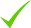IdentityEducation

Know how UrbanPro verifies Tutor details

Identity is verified based on matching the details uploaded by the Tutor with government databases.

## Overview

XYZ conducts classes in Math Olympiad. It is located in Naktala, Kolkata. It takes Regular Classes- at the Institute.

Naktala, Kolkata, India - 700047

## Verified Info

Report this Profile

Is this listing inaccurate or duplicate? Any other problem?

Type the letters as shown below *

Please enter the letters as show below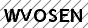Class Location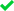At the Institute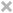Online (video chat via skype, google hangout etc)

## Reviews

this is test message this is test message this is test message this is test message this is test message this is test message this is test message

No Reviews yet! Be the first one to Review

## FAQs

1. Which classes do you teach?

2. Do you provide a demo class?

No, We don't provide a demo class.

3. Where are you located?

We are located in Naktala, Kolkata.

rs 150 per exam
Dislike Bookmark

Rs 300/hr
Dislike Bookmark

Rs 300/hr
Dislike Bookmark

Rs 300/hr For maths , 2 days per wk is enough...If 1.5 hrs per day , then it's 3 hrs / wk...So , 3600/- per month.For online , 3200/- is the charge.
Dislike Bookmark

CP=Rs 1400 , Loss%=15% By using formula, SP= = Rs = Rs = Rs = Rs 1190 OR (by using unitary method) SP=CP-Loss=Rs (100-15)=Rs 85 When CP is Rs 100 , SP is Rs 85 When CP is Rs 1400 , SP=Rs =Rs 1190 ...more

CP=Rs 1400 , Loss%=15%

By using formula,

SP=[{(100-Loss%)/100}*CP] = Rs[{(100-15)/100}*1400] = Rs [{85/100}*1400] = Rs [85*14] = Rs 1190

OR (by using unitary method)

SP=CP-Loss=Rs (100-15)=Rs 85

When CP is Rs 100 , SP is Rs 85

When CP is Rs 1400 , SP=Rs [(85/100)* 1400]

=Rs 1190

Dislike Bookmark

Class LocationAt the InstituteOnline (video chat via skype, google hangout etc)

this is test message this is test message this is test message this is test message this is test message this is test message this is test message

No Reviews yet! Be the first one to Review

rs 150 per exam
Dislike Bookmark

Rs 300/hr
Dislike Bookmark

Rs 300/hr
Dislike Bookmark

Rs 300/hr For maths , 2 days per wk is enough...If 1.5 hrs per day , then it's 3 hrs / wk...So , 3600/- per month.For online , 3200/- is the charge.
Dislike Bookmark

CP=Rs 1400 , Loss%=15% By using formula, SP= = Rs = Rs = Rs = Rs 1190 OR (by using unitary method) SP=CP-Loss=Rs (100-15)=Rs 85 When CP is Rs 100 , SP is Rs 85 When CP is Rs 1400 , SP=Rs =Rs 1190 ...more

CP=Rs 1400 , Loss%=15%

By using formula,

SP=[{(100-Loss%)/100}*CP] = Rs[{(100-15)/100}*1400] = Rs [{85/100}*1400] = Rs [85*14] = Rs 1190

OR (by using unitary method)

SP=CP-Loss=Rs (100-15)=Rs 85

When CP is Rs 100 , SP is Rs 85

When CP is Rs 1400 , SP=Rs [(85/100)* 1400]

=Rs 1190

Dislike Bookmark

XYZ conducts classes in Math Olympiad. It is located in Naktala, Kolkata. It takes Regular Classes- at the Institute.

Recommended Profiles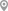Rahara, KolkataBARASAT, KolkataHaridevpur, KolkataSonarpur, KolkataMotijhil, KolkataMotijhil, Kolkata

X

Let us shortlist and give the best tutors and institutes.

or

Send Enquiry to XYZ

Let XYZ know you are interested in their class